# Keywords and Formulas

### Simple Statistics

The base SAS procedures use a standardized set of keywords to refer to statistics. You specify these keywords in SAS statements to request the statistics to be displayed or stored in an output data set.

In the following notation, summation is over observations that contain nonmissing values of the analyzed variable and, except where shown, over nonmissing weights and frequencies of one or more:

x i

• is the nonmissing value of the analyzed variable for observation i .

f i

• is the frequency that is associated with if you use a FREQ statement. If you omit the FREQ statement, then f i =1 for all i .

w i

• is the weight that is associated with x i if you use a WEIGHT statement. The base procedures automatically exclude the values of x i with missing weights from the analysis.

By default, the base procedures treat a negative weight as if it is equal to zero. However, if you use the EXCLNPWGT option in the PROC statement, then the procedure also excludes those values of x i with nonpositive weights. Note that most SAS/STAT procedures, such as PROC TTEST and PROC GLM, exclude values with nonpositive weights by default.

If you omit the WEIGHT statement, then w i =1 for all i .

n

• is the number of nonmissing values of x i , ˆ‘ f f i . If you use the EXCLNPWGT option and the WEIGHT statement, then is the number of nonmissing values with positive weights.

x

• is the mean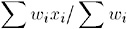s 2

• is the variance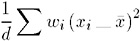where d is the variance divisor (the VARDEF= option) that you specify in the PROC statement. Valid values are as follows :

When VARDEF=

d equals

N

n

DF

n __1

WEIGHT

ˆ‘ w i

WDF

ˆ‘ w i __1

The default is DF.

z i

• is the standardized variable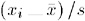The standard keywords and formulas for each statistic follow. Some formulas use keywords to designate the corresponding statistic.

Table A1.1: The Most Common Simple Statistics

Statistic

PROC MEANS and SUMMARY

PROC UNIVARIATE

PROC TABULATE

PROC REPORT

PROC CORR

PROC SQL

Number of missing values

X

X

X

X

X

Number of nonmissing values

X

X

X

X

X

X

Number of observations

X

X

X

Sum of weights

X

X

X

X

X

X

Mean

X

X

X

X

X

X

Sum

X

X

X

X

X

X

Extreme values

X

X

Minimum

X

X

X

X

X

X

Maximum

X

X

X

X

X

X

Range

X

X

X

X

X

Uncorrected sum of squares

X

X

X

X

X

X

Corrected sum of squares

X

X

X

X

X

X

Variance

X

X

X

X

X

X

Covariance

X

Standard deviation

X

X

X

X

X

X

Standard error of the mean

X

X

X

X

X

Coefficient of variation

X

X

X

X

X

Skewness

X

X

X

Kurtosis

X

X

X

Confidence Limits

of the mean

X

X

X

of the variance

X

of quantiles

X

Median

X

X

X

X

X

Mode

X

Percentiles/Deciles/ Quartiles

X

X

X

X

t test

for mean=0

X

X

X

X

X

for mean= ¼

X

Nonparametric tests for location

X

Tests for normality

X

Correlation coefficients

X

Cronbach s alpha

X

### Descriptive Statistics

The keywords for descriptive statistics are

CSS

• is the sum of squares corrected for the mean, computed as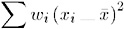CV

• is the percent coefficient of variation, computed as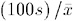KURTOSIS KURT

• is the kurtosis, which measures heaviness of tails . When VARDEF=DF, the kurtosis is computed as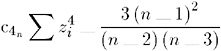where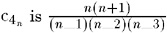. The weighted kurtosis is computed as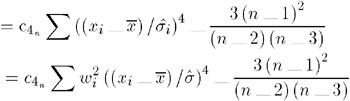When VARDEF=N, the kurtosis is computed as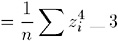and the weighted kurtosis is computed as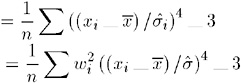where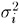is ƒ 2 / w i . The formula is invariant under the transformation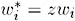, z > 0. When you use VARDEF=WDF or VARDEF=WEIGHT, the kurtosisis set to missing.

Note: PROC MEANS and PROC TABULATE do not compute weighted kurtosis.

MAX

• is the maximum value of x i .

MEAN

• is the arithmetic mean x .

MIN

• is the minimum value of x i .

MODE

• is the most frequent value of x i .

N

• is the number of values that are not missing. Observations with f i less than one and w i equal to missing or w i 0 (when you use the EXCLNPWGT option) are excluded from the analysis and are not included in the calculation of N.

NMISS

• is the number of x i values that are missing. Observations with less than one and equal to missing or w i 0 (when you use the EXCLNPWGT option) are excluded from the analysis and are not included in the calculation of NMISS.

NOBS

• is the total number of observations and is calculated as the sum of N and NMISS. However, if you use the WEIGHT statement, then NOBS is calculated as the sum of N, NMISS, and the number of observations excluded because of missing or nonpositive weights.

RANGE

• is the range and is calculated as the difference between maximum value and minimum value.

SKEWNESS SKEW

• is skewness, which measures the tendency of the deviations to be larger in one direction than in the other. When VARDEF=DF, the skewness is computed as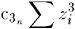• where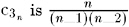. The weighted skewness is computed as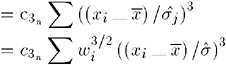• When VARDEF=N, the skewness is computed as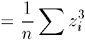• and the weighted skewness is computed as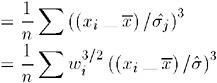• The formula is invariant under the transformation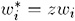, z > 0. When you use VARDEF=WDF or VARDEF=WEIGHT, the skewnessis set to missing.

• Note: PROC MEANS and PROC TABULATE do not compute weighted skewness.

STDDEVSTD

• is the standard deviation s and is computed as the square root of the variance, s 2 .

STDERR STDMEAN

• is the standard error of the mean, computed as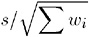• when VARDEF=DF, which is the default. Otherwise , STDERR is set to missing.

SUM

• is the sum, computed as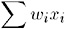SUMWGT

• is the sum of the weights, W , computed as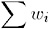USS

• is the uncorrected sum of squares, computed as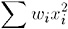VAR

• is the variance s 2 .

### Quantile and Related Statistics

The keywords for quantiles and related statistics are

MEDIAN

• is the middle value.

P1

• is the 1 st percentile.

P5

• is the 5 th percentile.

P10

• is the 10 th percentile.

P90

• is the 90 th percentile.

P95

• is the 95 th percentile.

P99

• is the 99 th percentile.

Q1

• is the lower quartile (25 th percentile).

Q3

• is the upper quartile (75 th percentile).

QRANGE

• is interquartile range and is calculated as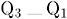You use the QNTLDEF= option (PCTLDEF= in PROC UNIVARIATE) to specify the method that the procedure uses to compute percentiles. Let n be the number of nonmissing values for a variable, and let x 1 , x 2 , , x n represent the ordered values of the variable such that is the smallest value, x 2 is next smallest value, and x n is the largest value. For the t th percentile between 0 and 1, let p = t /100. Then define as the integer part of np and g as the fractional part of np or ( n + 1) p , so that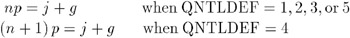Here, QNTLDEF= specifies the method that the procedure uses to compute the t th percentile, as shown in the table that follows.

When you use the WEIGHT statement, the t th percentile is computed aswhere w i is the weight associated with x i and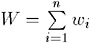is the sum of the weights.

When the observations have identical weights, the weighted percentiles are the same as the unweighted percentiles with QNTLDEF=5.

Table A1.2: Methods for Computing Quantile Statistics

QNTLDEF= Description

Formula

1

weighted average at x np

y = (1 ˆ’ g ) x j + gx j +1

where x o is taken to be x 1

2

observation numbered closest to np

y = x i

y = x j

y = x j + 1

if g ‰  1/2

if g = 1/2 and j is even and is even

if g = 1/2 j and is odd

where i is the integer part of np + 1/2

3

empirical distribution function

y = x j

y = x j +1

if g ‰

if g > 0

4

weighted average aimed at x ( n+1 ) p

y = (1 ˆ’ g ) x j + gx j + 1

where x n+1 is taken to be x n

5

empirical distribution function with averaging

y = 1/2( x j + x j +1)

y = x j + 1

if g = 0

if g > 0

### Hypothesis Testing Statistics

The keywords for hypothesis testing statistics are

T

• is the Student s t statistic to test the null hypothesis that the population mean is equal to ¼ and is calculated as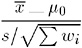• By default, ¼ is equal to zero. You can use the MU0= option in the PROC UNIVARIATE statement to specify ¼ . You must use VARDEF=DF, which is the default variance divisor, otherwise T is set to missing.

By default, when you use a WEIGHT statement, the procedure counts the values with nonpositive weights in the degrees of freedom. Use the EXCLNPWGT option in the PROC statement to exclude values with nonpositive weights. Most SAS/STAT procedures, such as PROC TTEST and PROC GLM automatically exclude values with nonpositive weights.

PROBT

• is the two-tailed p -value for Student s t statistic, T, with n ˆ’ 1 degrees of freedom. This is the probability under the null hypothesis of obtaining a more extreme value of T than is observed in this sample.

### Confidence Limits for the Mean

The keywords for confidence limits are

CLM

• is the two-sided confidence limit for the mean. A two-sided 100 (1 ˆ’ ± )percent confidence interval for the mean has upper and lower limits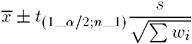where s is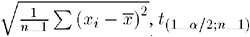is the (1 ˆ’ ± /2) critical value of the Student s t statistics with n ˆ’ 1 degrees of freedom, and ± is the value of the ALPHA= option which by default is 0.05. Unless you use VARDEF=DF, which is the default variance divisor, CLM is set to missing.

LCLM

• is the one-sided confidence limit below the mean. The one-sided 100(1 ˆ’ ± )percent confidence interval for the mean has the lower limit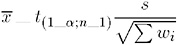• Unless you use VARDEF=DF, which is the default variance divisor, LCLM is set to missing.

UCLM

• is the one-sided confidence limit above the mean. The one-sided 100(1 ˆ’ ± )percent confidence interval for the mean has the upper limit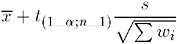• Unless you use VARDEF=DF, which is the default variance divisor, UCLM is set to missing.

### Using Weights

For more information on using weights and an example, see WEIGHT on page 63.

### Data Requirements for Summarization Procedures

The following are the minimal data requirements to compute unweighted statistics and do not describe recommended sample sizes. Statistics are reported as missing if VARDEF=DF (the default) and these requirements are not met:

• N and NMISS are computed regardless of the number of missing or nonmissing observations.

• SUM, MEAN, MAX, MIN, RANGE, USS, and CSS require at least one nonmissing observation.

• VAR, STD, STDERR, CV, T, and PRT require at least two nonmissing observations.

• SKEWNESS requires at least three nonmissing observations.

• KURTOSIS requires at least four nonmissing observations.

• SKEWNESS, KURTOSIS, T, and PROBT require that STD is greater than zero.

• CV requires that MEAN is not equal to zero.

• CLM, LCLM, UCLM, STDERR, T, and PROBT require that VARDEF=DF.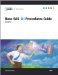Base SAS 9.1 Procedures Guide, Volumes 1, 2, 3 and 4
ISBN: 1590472047
EAN: 2147483647
Year: 2004
Pages: 142